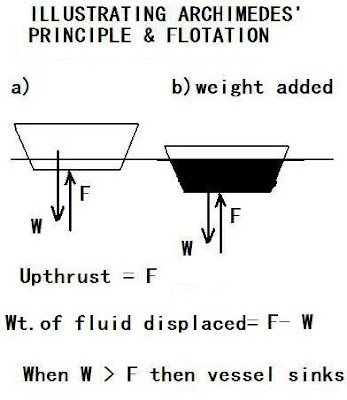## Sunday, November 14, 2010

### Can Fundies Be THIS Physics-ILLITERATE?It's amazing but one must ask this, as it's been brought to my attention that one fundie blogger insists that the putative (hypothetical) Noah's Ark would not have sunk with its tens of thousands of animal pairs, based on some balderdash he dreams up. But this can easily be disproven using elementary physics which this character obviously lacks.

One of the basic principles to be applied here is that of Archimedes, who reported yelped 'Eureka!' when he came up with it. He noticed on climbing into his bath that the water rose as he continued his immersion. He reasoned that the weight of the water displaced was equal to his own weight when fully immersed.

Thus, Archimedes' Principle, as stated in modern terms:

"A fluid acts on a body immersed in it with a net force that is vertically upward and equal in magnitude to the fluid displaced by the body".

The net force vertically upward (F- W) as shown (and equal to the weight of fluid displaced), is computed using the difference between the upthrust (F) and the weight (W). The vessel in the medium will always float provided that the upthrust exceeds the weight. Or, in more detailed terms: A body will sink if its own average density (total mass divided by total volume) exceeds the density of the liquid. In the case of salt water, this density is 1,100 kilograms per cubic meter. Thus, if the total density of a vessel (which includes all its ballast, added weight- divided by its volume) is greater than the density of the fluid on which it rests, it must sink. There are NO ifs, ands, or buts....since basic physics can't be violated.

Now, let's apply this principle to our previous analysis ( http://brane-space.blogspot.com/2010/11/m-brane-mailbag-more-letters-from.html), and use the maximum dimensions allowed for Noah's claimed Ark, assumed up to 550 feet long, 91.7 feet wide and 55 feet high. To make units consistent, we need to first convert all these to meters, then we will calculate the total effective volume - assuming the "ark' approximates to a prism of those dimensions. We know that 1 meter = 3.3 ft. so:

550' = 166.7 m

91.7' = 27.8 m

55' = 16.7

Then the total effective volume (which actually would be a maximum since a prism leaves no 'corners' out- giving fundies a tad more advantage) is:

V = (166.7m) x (27.8m) x (16.7m) = 77, 392 cubic meters

Thus, the maximum average density allowed would be (assuming salt water medium of the density above):

1,100 kg/ m^3

which again, is the density of the liquid in which the vessel is immersed.

Is this consistent with the total mass?

Recall we found the total mass for mammals, their excrement and food stocks alone came to M = 80,000,000 kg or 80,000 metric tons. Repeating this for reptiles, and using an average per pair mass here of 10.0 kg each, we found- when reckoning in all reptile pairs, their foodstuff mass (to keep them alive) + excrement = 402,000,000 kg or 402,000 metric tons.

So the total effective mammal + reptile pair mass (with ancillary wt. of food, feces) =

80,000,000 kg + 402,000,000 kg = 482,000,000 kg

The average density obtained is:

D = M/V = (482,000,000 kg) / 77, 392 m^3 = 6, 228 kg/ m^3

which clearly exceeds the density of sea water (at 1,100 kg/m^3) by a factor:

6, 228 kg/ m^3/ 1,100 kg/m^3 = 5.66

Thus, this vessel (Ark) must sink!

Now, a neat experiment readers can do on their own to show these principles. You will need only salt, a plastic soap dish and some lead shot (buck shot can also suffice).

Fill the sink or a large bowl with water and put the soap dish on it. Notice the flotation. Now, gradually add pieces of lead shot to the soap dish and note how it gradually sinks lower and lower - and (at some point) sinks, then too much shot is added. When does this happen? When the average density, e.g. mass of soap dish + lead shot, divided by the volume of the soap dish, exceeds the density of the fresh water (1 g/cm^3 or 1000 kg/ m^3).

The experiment can be repeated for salt water, and it will be found the initial float position of the soap dish is slightly higher, since salt water is more dense and confers a larger upthrust. You will also note that it takes a bit more shot to sink the dish - but like ALL vessels- at some point it must sink!

Archimedes' principle cannot be disobeyed! A point we wish some obtuse pastor bloggers would learn!